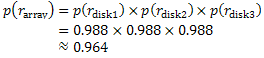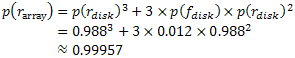### How Reliable is RAID?

We all know that when we want a highly available and reliable server we install a RAID solution, but how reliable actually is that? Well, obviously, you can work it out quite simply as we will see below, but before you do, you have to know what sort of RAID are you talking about, as some can be less reliable than a single disk. The most common types are RAID 0, 1 and 5. We will look at the reliability of each using real disks for the calculations, but before we do, let's recap on what the most common RAID types are.

### Common Types of RAID

RAID 0 is the Stripe set, which consists of 2 or more disks with data written in equal sized blocks to each of the disks. This is a fast way of reading and writing data to disk, but it gives you no redundancy at all. In fact, RAID 0 is actually less reliable than a single disk, as all the disks are in series from a reliability point of view. If you lose one disk in the array, you've lost the whole thing. RAID 0 is used purely to speed up disk access. If you have two disks your access will approach twice as fast; three disks are nearly three times as fast, etc. Actually, you don't quite achieve these performance gains due to overheads, etc.

RAID 5, on the other hand is the stripe set with parity, which can cope with one disk failing. You need a minimum of 3 disks to deploy RAID 5, and you will lose the capacity of one, that is to say that a 3-disk RAID 5 array using 0.5TB disks will have a capacity of 1TB, whereas the RAID 0 solution would have 1.5TB. Similarly, if we had a 5-disk RAID 5 array with the same disks as before then we would have 2TB at our disposal. The reason for this is that data is written in equal sized blocks to each of the disks as with RAID 0, but we write a parity block to one of the disks in each stripe. The diagram below explains. The parity is simply the bit wise Exclusive-OR of all the other blocks. It then becomes obvious that we can regenerate any one disk if it should fail by XORing all the remaining disks together. However, if we lose more than one disk, we've lost the lot again. RAID 5 arrays are also faster than single disk solutions, as we can read from and write to several disks at once, but due to the parity data, it will be slower than RAID 0.

Finally, we have RAID 1, which is the mirror set and consists of two identical disks. All data is written to both disks, so from a reliability point of view they are in parallel and the array is accessible with only one disk working. This means that mirroring two 500GB disks will only give 500GB of storage. You can read from both at once, but as you have to write to both at the same time this is slightly slower than a single disk due to the overheads. However, we can again cope with one disk failure. The power of this technique comes into its own when we start combining RAID arrays, e.g. we can mirror two RAID 5 arrays to cope with total failure of one array and a single disk failure in the other.

### Reliability of Disks

In order to calculate the reliability and availability of our RAID array we need to know how reliable our disks are. Manufacturers can quote this in one of two ways: Failure Rate or Mean Time To Failure (MTTF). These figures can sometimes seem misleading, so we'll look at how they're related and what they actually mean in terms of reliability of your disk arrays. Actually, the MTTF and Failure Rate are related via a simple calculation, as the annualised failure rate is usually quoted, which is simply the percentage of disks that will fail in a particular year given the MTTF of the drives.

If we take an actual drive as an example, the Seagate Barracuda 500GB 32MB Cache ST3500320AS, it has a stated MTTF of 750,000 hours or around 85.6 years. This doesn't mean that the drive will actually last that long, it means that on average in 750,000 disk drive hours you will get one failure. So, if we have 1000 disks in our data centre then we will on average suffer a failure every 750 hours or one disk will fail every month, on average (the 'on average' is important, as you could suffer three disk failures this month and none for the next two, for example). These figures are arrived at by the manufacturers in exactly that way. They will run a set of disks (usually under heavy load and probably at high temperature) for a set time and look at how many failed. For example, if they ran 2,000 disks for a month and had 2 failures, then they would get (2000 x 744)/2 = 744,000 hours (assuming a 31-day month as 31 x 24=744).

So how does this relate to the annualised failure rate? Quite simply, if we have one disk failure every 750,000 hours what percentage fail in one year? The first step is to work out how many years 750,000 hours is, so we have 750,000/(24 x 365.25), which is approximately 85.6 years. To get the annualised failure rate we take the inverse, i.e. 1/85.6, which gives a failure rate of nearly 1.2%. Of course, you have to remember that these figures do not take into account any batch failures, i.e. a fault in manufacturing causing a whole batch of disks to be faulty or less reliable.

### Calculating the Reliability of RAID

We can now use the annualised failure rate of the disks from the previous section to calculate the reliability of a RAID array. We will look at several scenarios to see how reliable, or not, the common types of RAID are. We will start with a 3-disk RAID 0 solution. Each disk has an annualised failure rate of 1.2% or a probability of failing of 0.012, which gives us a probability of 0.988 that the drive will still be running at the end of the year. Now a RAID 0 array has all the disks in series, i.e. all the disks must be working for the array to work. If any one disk fails then we have lost the whole array. Therefore, we have the probability of reliability:So, we can see from this that a RAID 0 array might be faster than a single disk, but it is less reliable. OK, we knew that RAID 0 gave us no redundancy, so what if we look at RAID 1 or RAID 5? Which one of these is more reliable? Let's look at a 2-disk RAID 1 array (it can only really be 2-disk remember). In this case the drives are in parallel, so it will only fail if both drives fail; it will still work if either one or the other or both drives are still working. Therefore, we have the probability of reliability:RAID 1 is clearly much more reliable than RAID 0 and a single disk solution. Now we'll look at a 3-disk RAID 5 solution. The complication here is that it isn't simply in parallel or series, the array will keep working in the event of any one disk failure or no failures. The easiest way to look at this is to add up all the probabilities of the situations where it is still running. A table makes it easy to see.You can see from the table that we are interested in the first three rows, i.e. when the array is still working. We can now simply add these up to get the overall reliability for the array as follows:So, we can see that this is much more reliable than a single disk solution, but less reliable than RAID 1. Indeed, the more disks you have in a RAID 5 array, the less reliable it becomes. In fact, a 5-disk RAID 5 array will have a reliability of around 0.99859. At what point does RAID 5 become less reliable than a single disk? If you work it out, it turns out that in this case a 14-disk RAID 5 array has almost the identical reliability to a single disk. Of course, you have much more storage and faster access, but no more reliability.
What if we were to combine these arrays, e.g. mirror a RAID 0 stripe set? Well, it's simply a matter of combining the reliabilities of each RAID 0 array in parallel. The reliability of a 3-disk RAID 0 array was approximately 0.964. If we now put this figure into the RAID 1 calculation above instead of the 0.988 disk reliability, this will give us a reliability of approximately 0.9987, more reliable than a 5-disk RAID 5 array. Of course, if we mirror a 3-disk RAID 5 array, then we would get a reliability of approximately 0.99999982 - very reliable.
The important thing here is to be able to interpret what manufacturers are saying and predict failures. To be able to do this you have to know how to calculate the reliability of your system. If you have 100 servers with 5-disk RAID 5 arrays in them, how many system failures will you get in a 5-year operating life cycle and how many disks will you need to replace? Disk replacement is simple, you have 500 disks in total with a MTTF of 750,000 hours, or one failure every 1500 hours, which is one failure every 62.5 days. If the life span of your data centre is 5 years, then you will get around 29 disk failures, but how many system failures? Well, the reliability of each system is 0.99859, which implies that the annualised failure rate for the system is 1 - 0.99859 = 0.00141. If this is the percentage of failures per year, then the MTTF will be 1/0.00141 which is approximately 709.22 years. We have 100 such systems, so we will get a failure in around 7 years 33 days. This means that we might well get 1 system failure during the 5-year life span, but we may not get any.